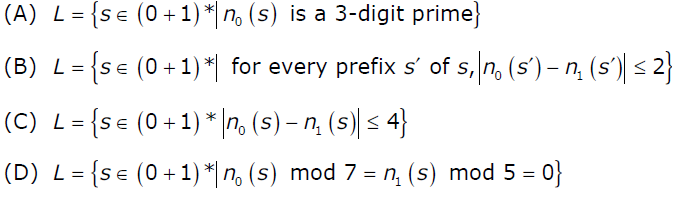# GATE | GATE-CS-2006 | Question 29

• Last Updated : 28 Jun, 2021

If s is a string over (0 + 1)* then let n0(s) denote the number of 0’s in s and n1(s) the number of 1’s in s. Which one of the following languages is not regular?7″ />
(A) A
(B) B
(C) C
(D) D

Explanation: Languages in option (A) And (D) are finite so both the options are eliminated.

For option A:
There are finite no. of 3 digit prime numbers. There exists a FA for every finite set. Hence FA is possible.

For option D:
Possible remainders for 7 is 0 to 6, and for 5 its 0 to 4. Using 35 states, FA can be made.

For option B:
We can have 6 states (including 1 reject state)
state 1: difference is 0
state 2: difference is 1 (more 1s)
state 3: difference is 1 (more 0s)
state 4: difference is 2 (more 1s)
state 5: difference is 2 (more 0s)
state 6: reject state for difference >= 3

Suppose the string is 000101
Scan 0 -> state 3
Scan 0 -> state 5
Scan 0 -> reject (since diff. is 3 now)

Similarly if we try for string: 010100, this will be accepted.

Quiz of this Question

My Personal Notes arrow_drop_up